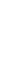# Drift scan methodBeam maps are produced from drift scans performed periodically on Cygnus A (CygA hereafter). Cyg A is chosen for the drift scans since it is one of the brightest compact radio sources in the northern sky, with a brightness of 1589 Jy (Birzan et al. 2004) an extent of approximately 5′ at 1.4 GHz, which makes it an unresolved continuum source for a single WSRT dish. During the drift scan measurement the PAF is at a fixed position on the sky and Cyg A drifts through the field of view in a straight line. The separation between the drifts is 0.1 degrees in declination. This is then repeated 31 times to cover the whole field of view of the 40 Apertif beams. Figure 1 illustrates this process.

Drift scan observations are scheduled using the aperdrift code : https://github.com/kmhess/aperdriftFigure 1. Illustration of drift scan observations. The dots represent the beam centres of the 40 Apertif beams, and the lines represent individual drifts across the field of view of the Apertif footprint.

## Beam models

The auto correlation data from the drift scans is used to construct fits images of each beams response to Cyg A. Then we perform a 40 times 40 pixel spline interpolation for each beam to minimise the distorting effect of bad data (e.g. RFI) to the beam map. For this, we use the scipy package interpolate.RectBivariateSpline, which performs a bivariate spline approximation over a rectangular mesh to smooth the data.

Once the fitting is done, we write out the resulting beam maps into a csv table and also into 9 times 40 fits files corresponding to 9 frequency bins for all 40 beams.

We divide the 150 MHz bandwidth into 10 frequency bins when extracting the auto correlation data from the drift scans. From these we construct beam models at 9 different frequencies (chan 1-9). We do not use the first frequency bin (chan 0) because it is always badly affected by RFI.

The code to produce the beam maps is available at: https://github.com/apertif/aperPBFigure 2. Beam maps for all 40 apertif beams reconstructed from drift scans. Contour levels are: 0.1, 0.2, 0.4, 0.5, 0.6, 0.8. Red contours highlight the 10% and the 50% sensitivity level. These drift scans were measured in September 2019 and channel 9 corresponds to a frequency of ~ 1.399 GHz.

## Time variability

Beam weights are measured at the start of every imaging observing run and are typically used for 2 weeks in a row. The beam weights define the shape of the compound beams. They depend on the quality of the beam weight measurement, (e.g. RFI at the time of the measurement) and also on the health of the system (e.g. broken elements on the PAFs, dysfunctional antennas). Drift scans are typically measured once per month due to the time intensive nature of the measurement. The beam models derived from drift scans observed at different times typically vary by a few percent (rms of the difference).Figure 3. Normalised distribution of the pixel by pixel difference between beam maps observed in September 2019 and in October 2019. The rms of the distribution is 0.018.

## Beam size change with frequency

Beam shapes and sizes change across the field of view of Apertif with the central beams being more symmetric and the beams along the edge of the field of view more elongated. Figures 4 and 5 show the average beam size (FWHM), and the FWHM along the x and y axis as a function of beam number. Figure 4 shows the beam size for frequency bin 7 (1.363 GHz) and Figure 5 shows the same for frequency bin 9 (1.399 GHz).Figure 4. FWHM as a function of beam number for channel 7 (1.363 GHz). The black line shows the average FWHM when fitting a 2D Gaussian function to the beam maps. The blue line shows the FWHM of the 2D Gaussian along the x-axis (r.a.) and the orange line shows the FWHM along the y-axis (dec). The shape of the CBs is not perfectly Gaussian, but a 2D Gaussian function is a good approximation for the beam shapes within a few percent.Figure 5. FWHM as a function of beam number for channel 9 (1.399 GHz). The black line shows the average FWHM when fitting a 2D Gaussian function to the beam maps. The blue line shows the FWHM of the 2D Gaussian along the x-axis (r.a.) and the orange line shows the FWHM along the y-axis (dec).

Beam sizes change linearly with frequency. The frequency dependence is on average: `-2.108e-08 · freq [Hz] + 63.47`. This is based on fitting a 2D Gaussian to each beam map at each frequency, taking the average FWHM from the 2D Gaussian fit and then fitting a first order polynomial to the FWHM vs. frequency for each bin. The results were then averaged for 14 different drift scan measurements. Figure 6 shows the average beam size for each 40 beams as a function of frequency bin for a set of drift scans (grey lines). The dashed black line shows the average fitted line to the data. Some of the beams occasionally show non smooth variation with the beam size (for example beam 7, see also in Figure 4, 5). The cause for this in most cases is due to the effect of RFI in certain frequency bins.Figure 6. FWHM of CBs as a function of frequency bins. We divide the 150 MHz bandwidth into 10 frequency bins when constructing the CB maps. The grey lines show the average FWHM from the 2D Gaussian fit to each CB, while the dashed black line shows the average fitted line (a and b are the parameters of the line).